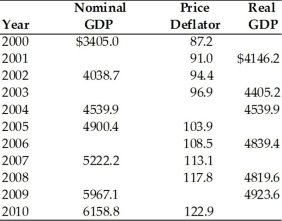• FAQ
• Contact/ Homework Answers / Economics / 31) The real value of an economic variable is A) the
Not my Question
Flag Content

# Question

31) The real value of an economic variable is

A) the consumer price index.

B) the producer price.

C) expressed in terms of actual market prices at which goods are sold.

D) computed by taking the nominal value and dividing by the appropriate price index.

32) Constant dollars are

A) the same as current dollars.

B) dollars corrected for general price level changes.

C) what nominal GDP is measured in.

D) when an individual does not receive a cost of living increase.

33) The values of variables such as GDP expressed in current dollars are referred to as

A) the price index.

B) a deflator.

C) nominal values.

D) real values.

34) Measurement of economic values after adjustments have been made for changes in the average of prices between years in known as

A) the price index.

B) a deflator.

C) nominal values.

D) real values.

35) Real GDP is

A) the nominal value of all real goods produced in the nation in a year.

B) GDP corrected for changes in the average of overall prices.

C) a misnomer since all GDP figures have to be in dollar values.

D) the value of output in current dollars.

36) Comparing two countries' nominal GDP over time is likely to be misleading if one wants to determine whether standards of living are better in one country because

A) the figures must be adjusted for different types of currency.

B) the figures must be adjusted for price changes and population differences.

C) the figures must be adjusted to account for production differences.

D) NDP instead of GDP should be used.37) Refer to the above table. The value of the GDP deflator in 2009 is

A) 121.2.

B) 122.1.

C) 122.9.

38) Refer to the above table. Between 2009 and 2010 real GDP

A) increased by \$191.7 billion.

B) increased by \$1.7 billion.

C) increased by \$87.6 billion.

D) increased by \$158.2 billion.

39) Refer to the above table. The nominal GDP for 2008 is

A) \$4819.6.

B) \$4091.3.

C) \$5677.5.

40) Refer to the above table. Real GDP for year 2007 was

A) \$4819.6 billion.

B) \$5222.2 billion.

C) \$4617.3 billion.